### Square Inscribed in a 12-Point Star

Illustration of a square inscribed in a closed concave geometric figure with 24 sides in the shape of…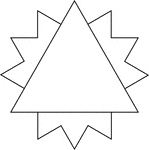### Triangle Inscribed In A 12-Point Star

Illustration of an equilateral triangle inscribed in a closed concave geometric figure with 24 sides…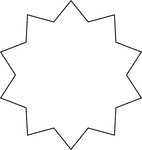### 10-Point Star

Illustration of a closed concave geometric figure with 20 sides in the shape of a 10-point star.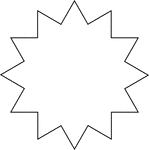### 12-Point Star

Illustration of a closed concave geometric figure with 24 sides in the shape of a 12-point star.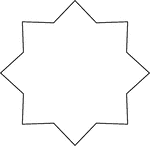### 8-Point Star

Illustration of a closed concave geometric figure with 16 sides in the shape of a 8-point star.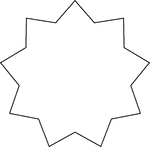### 9-Point Star

Illustration of a closed concave geometric figure with 18 sides in the shape of a 9-point star.